#### Bisection Method Calculator in Excel

The setup of the bisection method is about doing a specific task in Excel. With the bisection method, we are going to find the root of a given function.

The bisection method allows you to find the root of any function in a given search interval. In this Excel tutorial you will calculate guesses, middle point and error.

The method works by dividing the interval that contains the root into two equal parts and then determining which part of the interval contains the root. This process is repeated until the root is found with a desired degree of accuracy.

## Finding roots

The first step in creating a Bisection Method calculator in Excel is to define the function for which you want to find the root. This function should be entered into a cell in the Excel worksheet.

For example, suppose you want to find the root of the following function:

f(x) = 2e^2 -4x+2

Present the function and two possible roots.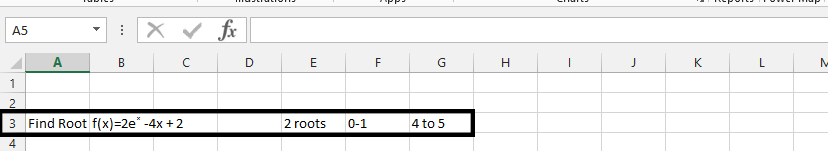Make some assumptions.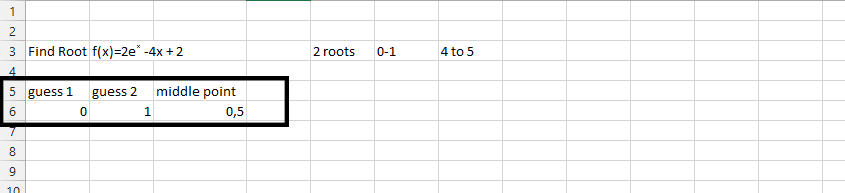Place three different roots beside the guesses.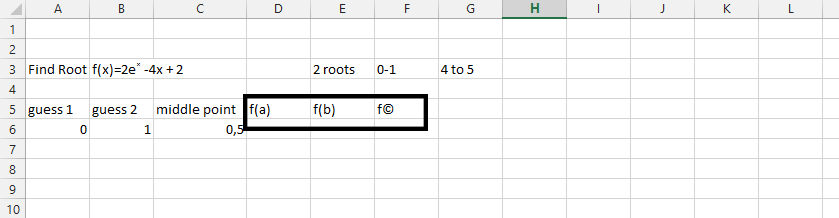In addition to F(c), enter the tolerance, which is the maximum acceptable error in the estimate of the root. This tolerance should be entered into a cell in the Excel worksheet.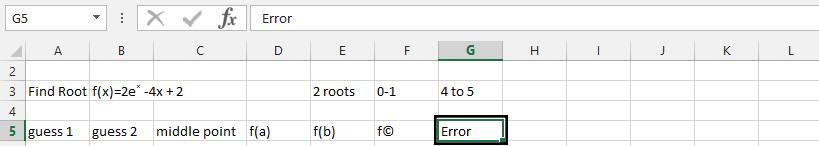Decide the value that should be accurate besides the error.

For example, suppose you want to find the root with a tolerance of 3. To define this tolerance in Excel, you can enter the value 3 into a cell, such as cell H5.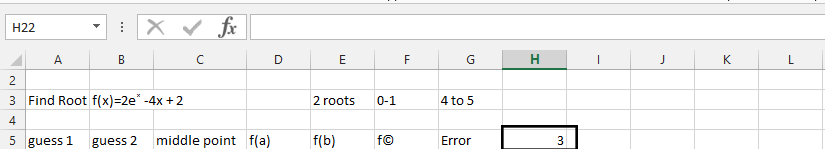## Calculating bisection method

The next step is to create the Bisection Method formula in Excel. This formula will be used to calculate the midpoint of the interval and to determine which part of the interval contains the root.

In the cell under f(a) (1), type in =2*exp(a6)-5*a6+2 (2).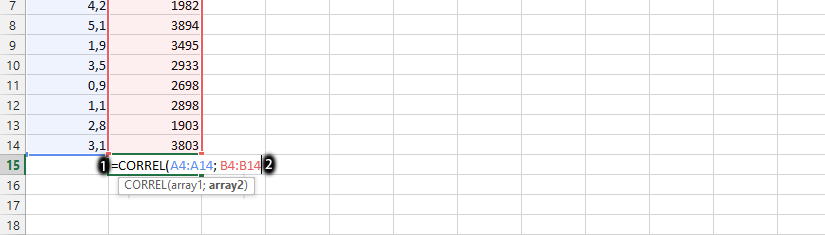Note: The 2 in front of the formula in this step is the one we placed at the beginning.

Drag the small square from f(a) to f(c).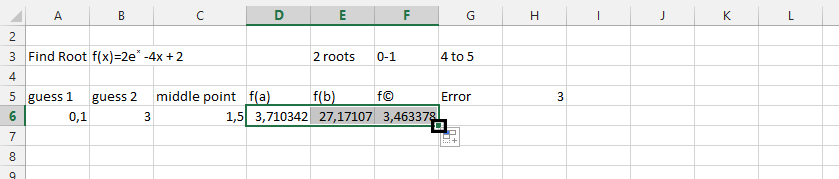Click on the cell below the error, type =ABS(B6), and then hit enter.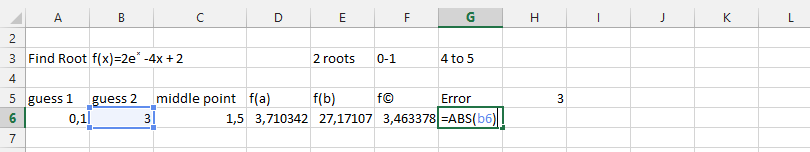Click under the cell with 3 in it (1), and type in =IF (G6=3;1(true);0(false)) (2), and then press enter.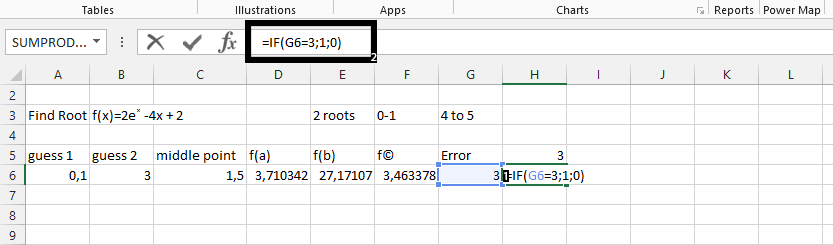Mark the row with values in it.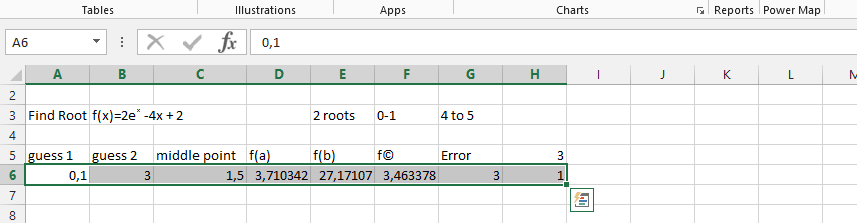Click on the small square showing on the right lower corner, and keep dragging it down until the value under 3 stably shows 1.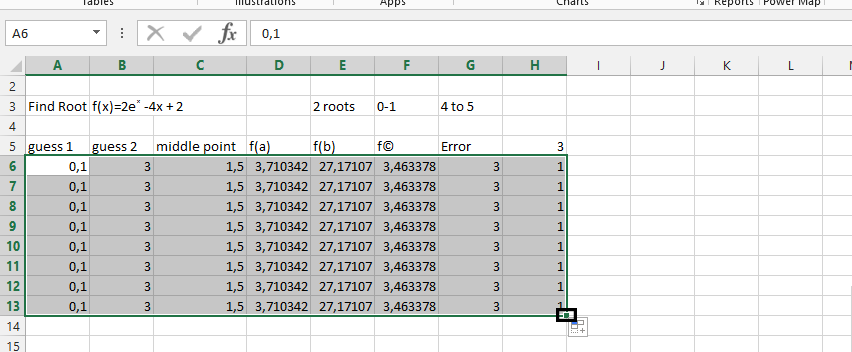The final step is to test the Bisection Method calculator in Excel. To test the calculator, simply enter the values for the function, interval, and tolerance into the appropriate cells.

The Bisection Method calculator in Excel will continue to repeat the Bisection Method formula until the root is found or until the tolerance is reached. The root of the function will be displayed in the “E” column.

The Bisection Method is a powerful numerical method used to find the root of a function. By creating a Bisection Method calculator in Excel, you can easily find the root of a function with a desired degree of accuracy. The Bisection Method calculator in Excel is simple to use and can be created with just a few formulas and a “Do Until” loop.